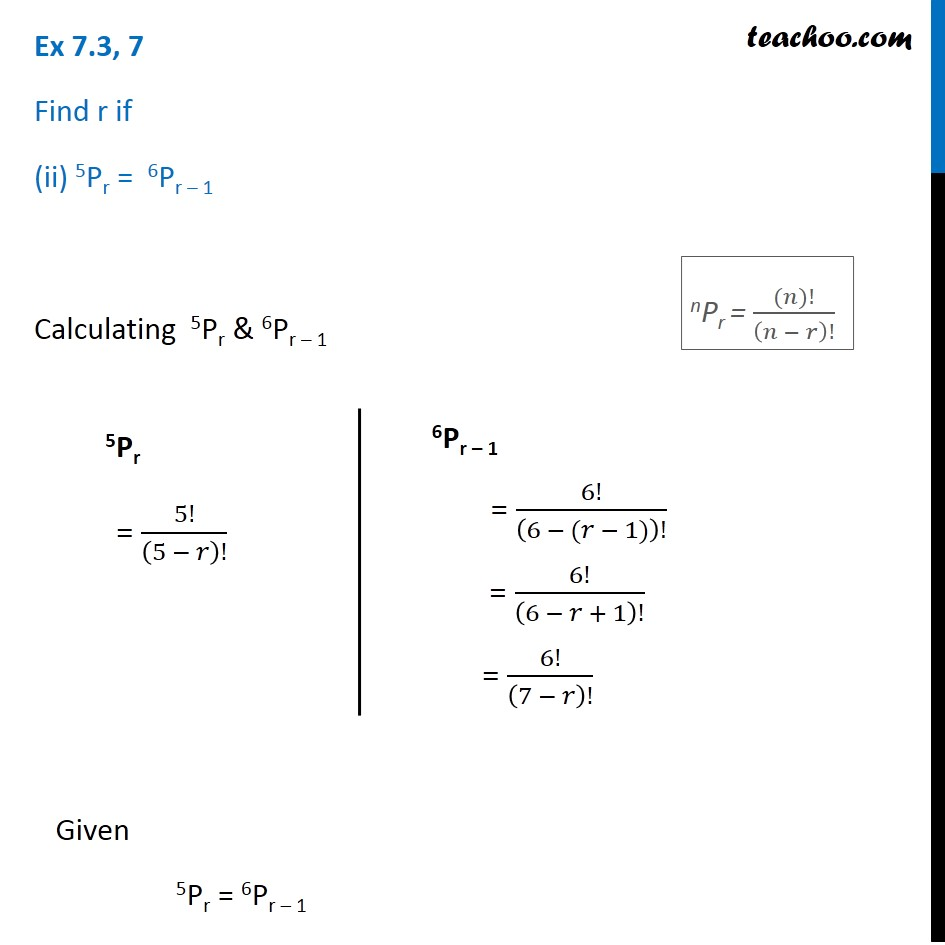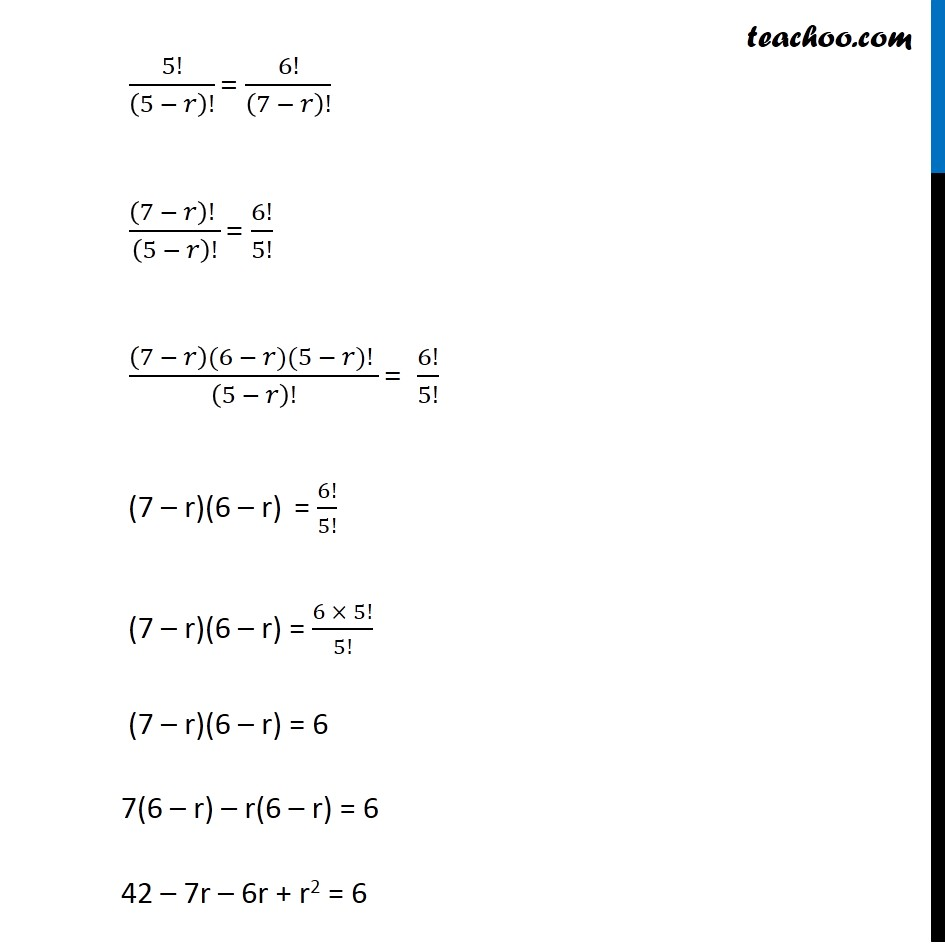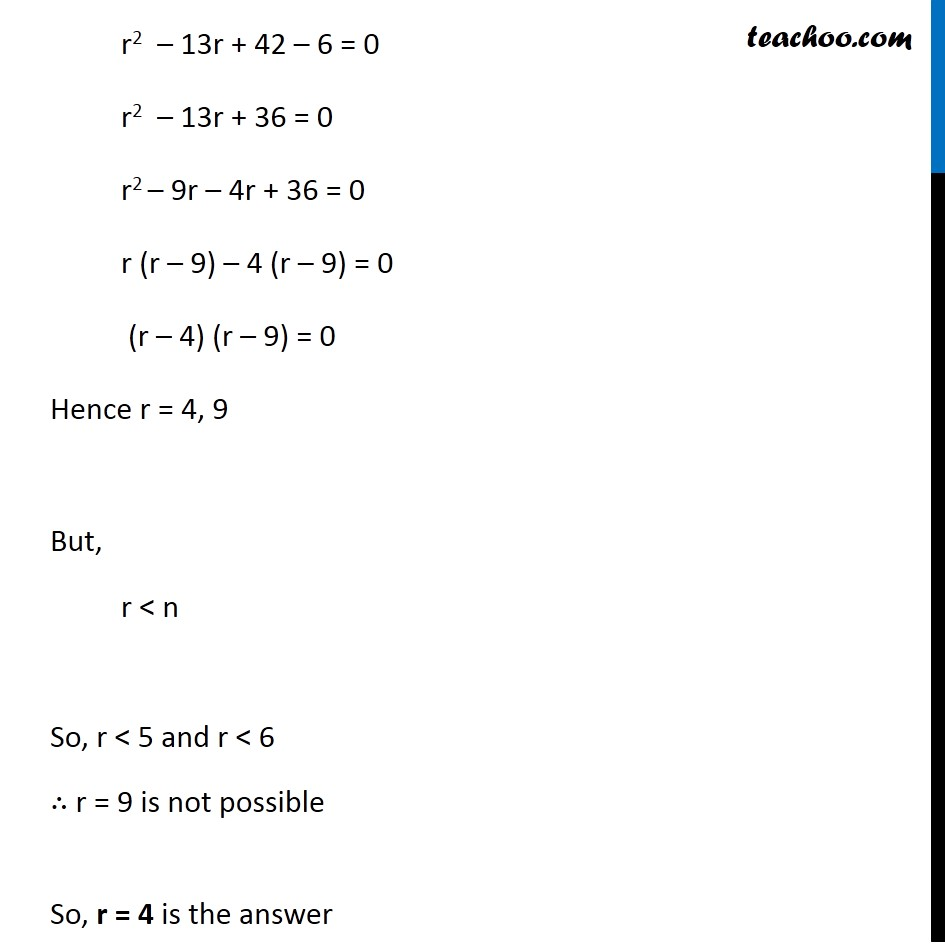Ex 6.3

Chapter 6 Class 11 Permutations and Combinations
Serial order wiseLearn in your speed, with individual attention - Teachoo Maths 1-on-1 Class

### Transcript

Ex 6.3, 7 Find r if (ii) 5Pr = 6Pr – 1 Calculating 5Pr & 6Pr – 1 Given 5Pr = 6Pr – 1 5Pr = 5!/(5 − 𝑟)! 6Pr – 1 = 6!/(6 − (𝑟 − 1))! = 6!/(6 − 𝑟 + 1)! = 6!/(7 − 𝑟)! nPr = ((𝑛)!)/(𝑛 − 𝑟)! 5!/(5 − 𝑟)! = 6!/(7 − 𝑟)! ((7 − 𝑟)! )/(5 − 𝑟)! = 6!/5! ((7 − 𝑟)(6 − 𝑟)(5 − 𝑟)! )/(5 − 𝑟)! = 6!/5! (7 – r)(6 – r) = 6!/5! (7 – r)(6 – r) = (6 × 5!)/5! (7 – r)(6 – r) = 6 7(6 – r) – r(6 – r) = 6 42 – 7r – 6r + r2 = 6 r2 – 13r + 42 – 6 = 0 r2 – 13r + 36 = 0 r2 – 9r – 4r + 36 = 0 r (r – 9) – 4 (r – 9) = 0 (r – 4) (r – 9) = 0 Hence r = 4, 9 But, r < n So, r < 5 and r < 6 ∴ r = 9 is not possible So, r = 4 is the answer